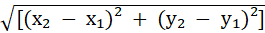# Distance Calculator

## Why use the online distance calculator?

You can easily and quickly calculate the distance between two points using MYcalcu’s distance calculator.

This calculator requires no payment or login restrictions. It’s 100% free.

## How does it work?

In order to find out the distance between two coordinates, this calculator uses the following formula:## How to use the distance calculator?

Enter the values in the required fields and click calculate to find out the distance between two points.